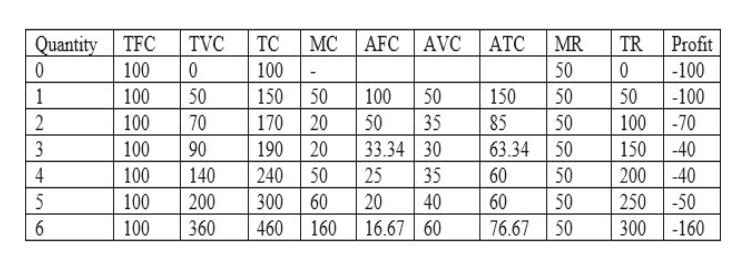# 4. Problems and Applications Q4Ball Bearings, Inc., faces costs of production as follows:QuantityTotal Fixed CostsTotal Variable Costs(Dollars)(Dollars)01000110050210070310090410014051002006100360 Complete the following table by calculating the company's total cost, marginal cost, average fixed cost, average variable cost, and average total cost at each level of production.QuantityTotal CostMarginal CostAverage Fixed CostAverage Variable CostAverage Total Cost(Dollars)(Dollars)(Dollars)(Dollars)(Dollars)0      1              2              3              4              5              6               The price of a case of ball bearings is \$50. Seeing that he can't make a profit, the company's chief executive officer (CEO) decides to shut down operations.The firm's profit in this case is ____________. (Note: If the firm suffers a loss, enter a negative number in this cell.) True or False: This was a wise decision.TrueFalse  Vaguely remembering his introductory economics course, the company's chief financial officer tells the CEO it is better to produce 1 case of ball bearings, because marginal revenue equals marginal cost at that quantity.At this level of production, the firm's profit is ____________. (Note: If the firm suffers a loss, enter a negative number in this cell.). True or False: This is the best decision the firm can make.TrueFalse

Question
409 views

## 4. Problems and Applications Q4

Ball Bearings, Inc., faces costs of production as follows:
Quantity
Total Fixed Costs
Total Variable Costs
(Dollars)
(Dollars)
0 100 0
1 100 50
2 100 70
3 100 90
4 100 140
5 100 200
6 100 360

Complete the following table by calculating the company's total cost, marginal cost, average fixed cost, average variable cost, and average total cost at each level of production.
Quantity
Total Cost
Marginal Cost
Average Fixed Cost
Average Variable Cost
Average Total Cost
(Dollars)
(Dollars)
(Dollars)
(Dollars)
(Dollars)
0

1

2

3

4

5

6

The price of a case of ball bearings is \$50. Seeing that he can't make a profit, the company's chief executive officer (CEO) decides to shut down operations.
The firm's profit in this case is ____________. (Note: If the firm suffers a loss, enter a negative number in this cell.)

True or False: This was a wise decision.
True
False

Vaguely remembering his introductory economics course, the company's chief financial officer tells the CEO it is better to produce 1 case of ball bearings, because marginal revenue equals marginal cost at that quantity.
At this level of production, the firm's profit is ____________. (Note: If the firm suffers a loss, enter a negative number in this cell.).

True or False: This is the best decision the firm can make.
True
False
check_circle

Step 1

The following table can be completed and written as follows by using the following formulas:

1. TC = TFC + TVC
2. MCn + TCn – TCn-1
3. AFC = TFC / Quantity
4. AVC = TVC / Quantity
5. ATC = AFC + AVC
6. TR = Price * Quantity
7. Profit = TR – TChelp_outlineImage TranscriptioncloseQuantity TFC AFC AVC TC TVC MC АТС MR TR Profit -100 10 100 0 100 50 1 100 50 150 50 100 50 150 50 50 -100 10070 150-40 200 40 25050 300 -160 2 35 50 100 70 170 20 50 85 190 20 240 33.34 30 90 3 100 63.34 50 100 4 140 50 25 35 60 50 5 300 100 100 200 60 20 40 60 50 76.67 50 16.67 60 460 160 6 360 fullscreen
Step 2

Now, it is assumed that price of ball bearings is \$50 and looking at this level, it is foresure that the firm can’t make a profit. Hence, the CEO decides to shut-down its operations; therefore, the firm’s profit in this case is -\$100 as only fixed cost is ...

### Want to see the full answer?

See Solution

#### Want to see this answer and more?

Solutions are written by subject experts who are available 24/7. Questions are typically answered within 1 hour.*

See Solution
*Response times may vary by subject and question.
Tagged in